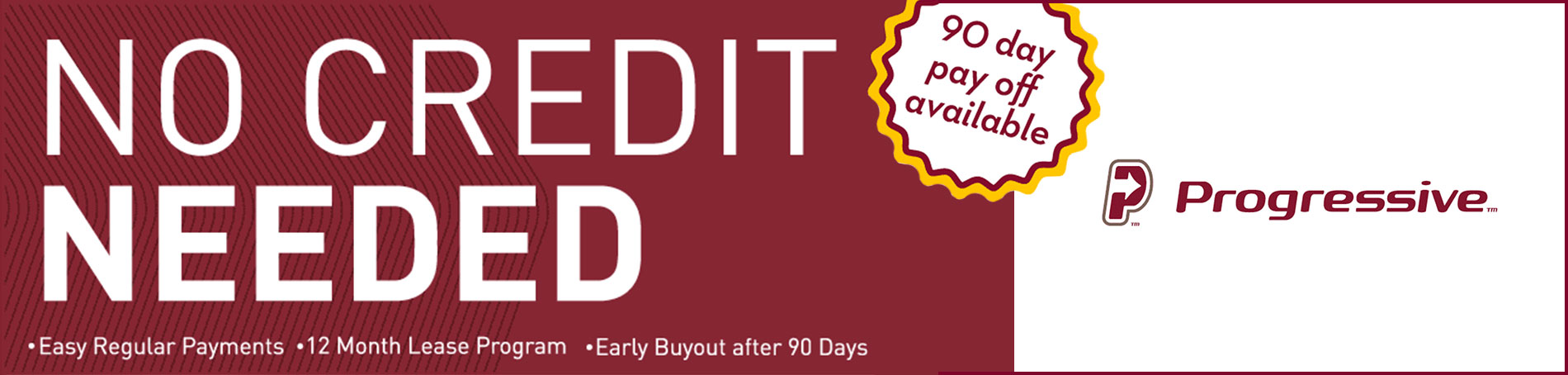Was: \$2,648.94
Now: \$2,012.94

Was: \$793.94
Now: \$687.94

Was: \$1,376.94
Now: \$1,058.94

Was: \$1,970.54
Now: \$1,546.54

Was: \$740.94
Now: \$617.86

Was: \$1,694.94
Now: \$1,482.94

Was: \$295.74
Now: \$279.72

Was: \$94.34
Now: \$62.54

Was: \$201.39
Now: \$157.94

Was: \$73.14
Now: \$51.94

Was: \$104.94
Now: \$76.32

Was: \$1,853.94
Now: \$1,217.94

Was: \$773.79
Now: \$667.79

Was: \$316.94
Now: \$210.94

Was: \$1,164.94
Now: \$857.54

Was: \$157.94
Now: \$104.82

\$846.94

Was: \$316.94
Now: \$210.94

Was: \$306.34
Now: \$200.34

Was: \$316.94
Now: \$210.94

Was: \$1,164.94
Now: \$857.54

Was: \$1,164.94
Now: \$846.94

Was: \$1,376.94
Now: \$1,058.94

Was: \$2,648.94
Now: \$2,012.94

Was: \$2,648.94
Now: \$2,012.94

Was: \$2,012.94
Now: \$1,482.94